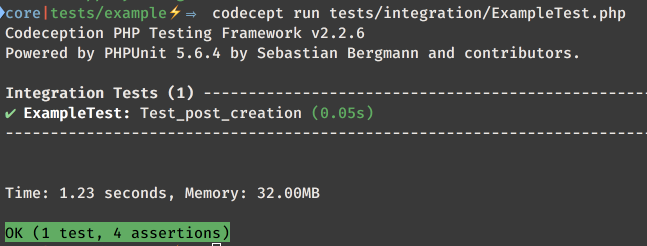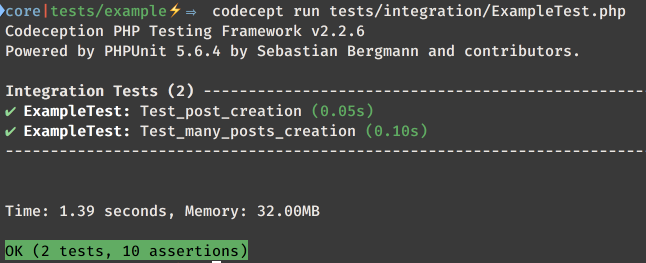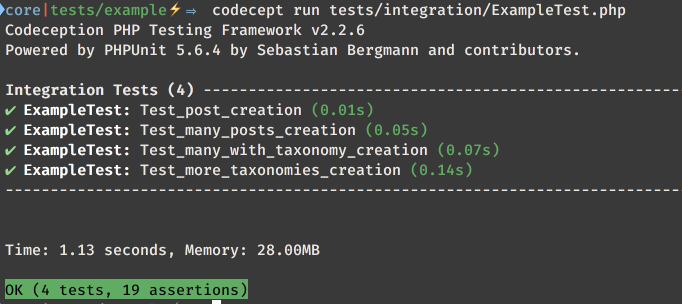# Using the post factory in WordPress integration tests

Zoom on the integration tests workhorse.

## Introduction

I’ve given a panoramic of the tools available to WordPress integration tests in a previous article and gone into the specifics of multisite in a secondo one.
With some knowledge of the context and the tools this post will be easy to understand but, just in case, the two posts above could provide some missing knowledge.
The context of the test methods is a test case extending either the base test case provided by the WordPress Core automated testing suite or a test case scaffolded using wp-browser wpcept command like

wpcept generate:wpunit integration Example

The empty tests case code should look like this:

<?php

class ExampleTest extends \Codeception\TestCase\WPTestCase
{
public function setUp()
{
// before
parent::setUp();

// your set up methods here
}

public function tearDown()
{
// your tear down methods here

// then
parent::tearDown();
}

// <<< any code from the example should replace this comment
}

When I run the tests I will do using Codeception CLI tool:

codecept run integration

The whole integration suite only contains one test case but should that not be true then, using Codeception, it’s true to run just one test:

codecept run tests/integration/ExampleTest.php

Since the Core suite uses the PhpUnit runner [PHPUnit own configuration guide](!g phpunit cli runner) will apply.
The differences between the two starting points are, at this stage, irrelevant.
I will, in this post, focus on the fundamental post factory provided by the test case.

## Creating a post

While I’ve already showed this in previous posts it’s worth taking a look at what a tester does not have to do when using the post factory.

public function test_post_creation()
{

$id = wp_insert_post(['post_title'=>'Some Post']);$post = get_post($id);$this->assertInstanceOf(WP_Post::class, $post);$this->assertEquals('post',$post->post_type);$factoryId = $this->factory()->post->create();$factoryPost = get_post($factoryId);$this->assertInstanceOf(WP_Post::class, $factoryPost);$this->assertEquals('post', $factoryPost->post_type); }In this case the difference between creating a post for testing purposes with a direct call to wp_inser_post or using the factory create method is not that notable. The reason I prefer to use the factory in the case of a single post too is that I can skip the post_title argument too, required by the wp_insert_post function, not leaving any doubt about the fact that the test is not testing posts with a specific post title. The difference becomes notable when creating multiple posts. public function test_many_posts_creation() {$count = 5;

$ids = array_map(function ($n) {
return wp_insert_post(['post_title' => 'Post ' . $n, 'post_status' => 'publish']); }, range(1,$count));

$this->assertCount(5,$ids);
$this->assertContainsOnly('int',$ids);
$this->assertCount(5, get_posts(['numberposts' => -1]));$factoryIds = $this->factory()->post->create_many($count);

$all = get_posts(['numberposts' => -1, 'fields' => 'ids']);$this->assertCount(10, $all);$this->assertEqualSets($all, array_merge($ids, $factoryIds)); }The test case above underlines a difference worth noting: when using the wp_insert_post function the default post_status is draft; this means that for the following get_posts call to return something I had to explicitly set the post status of each post to publish; this detail is often overlooked and cause of many headaches trying to find out why some trivial tests are failing. In the last line of the test case I’m using the assertEqualSets method to make sure the two sets are equals no matter the order; this is not a PHPUnit method but one provided by the WP_UnitTestCase class in the Core suite and the \Codeception\TestCase\WPTestCase for tests scaffolded by wp-browser; PHPUnit own assertEquals will take content and order into account. ## Taxonomies It’s been some time that the wp_insert_post and wp_update_post functions have allowed for a tax_input entry to specify one or more terms from one or more taxonomies to be assigned to the post at creation/update time. public function test_many_with_taxonomy_creation() {$taxonomy = 'some-taxonomy';
$term = 'some-term'; register_taxonomy($taxonomy, null);
wp_insert_term($term,$taxonomy);

$count = 5;$editor = $this->factory()->user->create(['role'=>'editor']);$this->assertTrue(user_can($editor,'edit_posts')); wp_set_current_user($editor);

$ids = array_map(function ($n) use ($taxonomy,$term) {
return wp_insert_post([
'post_title' => 'Post ' . $n, 'post_status' => 'publish', 'tax_input' => [$taxonomy => $term] ]); }, range(1,$count));

$tax_query = [ 'fields' => 'ids', 'numberposts' => -1, 'tax_query' => [ [ 'taxonomy' =>$taxonomy,
'terms' => $term, 'field' => 'slug' ] ] ];$this->assertCount(5, get_posts($tax_query));$factoryIds = $this->factory()->post->create_many($count,[
'tax_input' => [$taxonomy =>$term]
]);

$allIds = array_merge($ids, $factoryIds);$inDb = get_posts($tax_query);$this->assertCount(10, $inDb);$this->assertEqualSets($inDb,$allIds);
}Whether a tester is using wp_insert_post directory or the factory the operation of assigning a post taxonomy terms at creation time, will require the current user to be able to edit_posts (see the first article).
Beside that the post factory based approach keeps being smaller and clearer in terms of code than the one based on the wp_insert_post function.
Whatever the approach it’s important to note the peculiar syntax of the tax_input entry:

public function test_more_taxonomies_creation()
{
$flatTaxonomy = 'flat';$hierarchicalTaxonomy = 'hierarchical';

register_taxonomy($flatTaxonomy, 'post'); foreach (['flat1', 'flat2', 'flat3'] as$term) {
wp_insert_term($term,$flatTaxonomy);
}

register_taxonomy($hierarchicalTaxonomy, 'post', ['hierarchical' => true]);$hierarchicalTerms = [];
foreach (['hier1', 'hier2', 'hier3'] as $term) {$hierarchicalTerms[] = wp_insert_term($term,$hierarchicalTaxonomy);
}
$hierarchicalTerms = wp_list_pluck($hierarchicalTerms, 'term_id');

$count = 5;$editor = $this->factory()->user->create(['role' => 'editor']);$this->assertTrue(user_can($editor, 'edit_posts')); wp_set_current_user($editor);

array_map(function ($n) use ($flatTaxonomy, $hierarchicalTaxonomy,$hierarchicalTerms) {
return wp_insert_post([
'post_title' => 'Post ' . $n, 'post_status' => 'publish', 'tax_input' => [$flatTaxonomy => 'flat1, flat2, flat3',
$hierarchicalTaxonomy =>$hierarchicalTerms,
]
]);
}, range(1, $count));$flat1Posts = [
'fields' => 'ids',
'numberposts' => -1,
'tax_query' => [
[
'taxonomy' => $flatTaxonomy, 'terms' => 'flat1', 'field' => 'slug' ] ] ];$hier1Posts = [
'fields' => 'ids',
'numberposts' => -1,
'tax_query' => [
[
'taxonomy' => $hierarchicalTaxonomy, 'terms' => 'hier1', 'field' => 'slug' ] ] ];$this->assertCount(5, get_posts($flat1Posts));$this->assertCount(5, get_posts($hier1Posts));$factoryIds = $this->factory()->post->create_many($count, [
'tax_input' => [
$flatTaxonomy => 'flat1, flat2, flat3',$hierarchicalTaxonomy => $hierarchicalTerms ] ]);$this->assertCount(10, get_posts($flat1Posts));$this->assertCount(10, get_posts($hier1Posts)); }What’s going on in this test is that I’m registering a hierarchical and non-hierarchical taxonomy (flat) and creating 5 posts with each of the methods seen above passing the tax_input array directly. To note here is the different format used to pass the taxonomy terms: • for a non-hierarchical taxonomy the value should be a **comma separated list of taxonomy term slugs” • for a hierarchical taxonomy the value should instead be an array of term IDs This point, a requirement inherited from the wp_insert_method, is often forgotten in tests leading to much time wasting in search of a bug. ## Meta Last piece is inserting a post meta values at creation time: public function test_meta_insertion() {$id = wp_insert_post([
'post_title' => 'Post',
'post_status' =>'publish',
'meta_input' => [
'one' => 'foo-bar',
'two' => ['foo', 'bar'],
]
]);

$this->assertNotEmpty(get_post_meta($id, 'one'));
$this->assertNotEmpty(get_post_meta($id, 'two'));

$this->assertInternalType('string', get_post_meta($id, 'one', true));
$this->assertEquals(['foo', 'bar'], get_post_meta($id, 'two', true));
$this->assertEquals([['foo', 'bar']], get_post_meta($id, 'two', false));

$this->assertCount(1, get_posts(['meta_key' => 'one', 'meta_compare' => 'EXISTS']));$this->assertCount(1, get_posts(['meta_key' => 'two', 'meta_compare' => 'EXISTS']));
$this->assertCount(1, get_posts(['meta_key' => 'two', 'meta_value' => serialize(['foo', 'bar'])]));$this->assertEmpty(get_posts(['meta_key' => 'two', 'meta_value' => 'foo']));
$this->assertEmpty(get_posts(['meta_key' => 'two', 'meta_value' => 'bar']));$factoryId = $this->factory()->post->create([ 'meta_input' => [ 'one' => 'foo-bar', 'two' => ['foo', 'bar'], ] ]);$this->assertNotEmpty(get_post_meta($factoryId, 'one'));$this->assertNotEmpty(get_post_meta($factoryId, 'two'));$this->assertInternalType('string', get_post_meta($factoryId, 'one', true));$this->assertEquals(['foo', 'bar'], get_post_meta($factoryId, 'two', true));$this->assertEquals([['foo', 'bar']], get_post_meta(\$factoryId, 'two', false));
}This last test shows once again how easy it is to attach meta to the posts while inserting them using the post factory or a wp_insert_post based approach.
It also shows a limit of both methods imposed, once again, from the wp_insert_post function: meta values set to array will not generate each a separated database entry but will be, instead, serialized.
The central block of assertions makes that clear.

## Next

I will give some space, next, to how the post factory works with custom post types and pages.